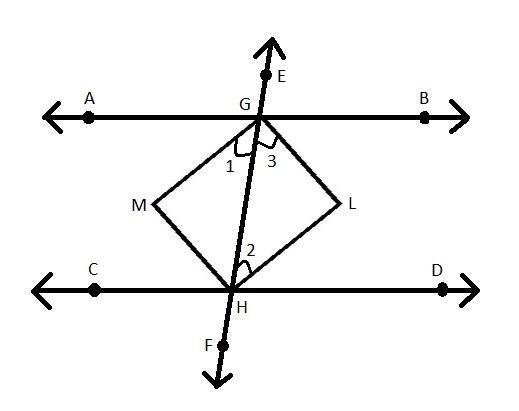QUESTION

# If two parallel lines are intersected by a transversal, then prove that bisectors of the interior angles form a rectangle.

Hint: First of all, construct a quadrilateral with two parallel lines intersected by a transversal and the bisectors of the interior angles. Then show that the formed quadrilateral is parallelogram and one of its angles is the right angle then the given quadrilateral is a rectangle.

Construction: Two parallel lines AB and CD and a transversal EF intersect them at G and H respectively. GM, HM, GL and HL are the bisectors of the two pairs of interior angles.To prove: GHML is a rectangle.
Proof:
Since $AB\parallel CD$
$\angle AGH = \angle DHG{\text{ }}\left( {{\text{Alternate interior angles}}} \right)$
Dividing both side by 2, we have
$\Rightarrow \dfrac{1}{2}\angle AGH = \dfrac{1}{2}\angle DHG \\ \Rightarrow \angle 1 = \angle 2{\text{ }}\left( {{\text{GM & HL are bisectors of }}\angle {\text{AGH and }}\angle {\text{DHG respectively}}} \right) \\ \Rightarrow GM\parallel HL{\text{ }}\left( {\angle 1{\text{ and }}\angle {\text{2 from a pair of alternate interior angles are equal}}} \right) \\$
Similarly, $GL\parallel MH$
So, GMHL is a parallelogram.
Since $AB\parallel CD$
We have $\angle BGH + \angle DHG = {180^\circ}$ as the sum of interior angles on the same side of the transversal is equal to ${180^\circ}$.
Dividing both sides by 2, we have
$\Rightarrow \dfrac{1}{2}\angle BGH + \dfrac{1}{2}\angle DHG = {90^\circ} \\ \Rightarrow \angle 3 + \angle 2 = {90^\circ}........................................................\left( 1 \right) \\$
In $\Delta GLH$ as the sum of the angles is equal to ${180^\circ}$, we have
$\Rightarrow \angle 2 + \angle 3 + \angle L = {180^\circ} \\ \Rightarrow {90^\circ} + \angle L = {180^\circ}{\text{ }}\left( {{\text{Using }}\left( 1 \right)} \right) \\ \Rightarrow \angle L = {180^\circ} - {90^\circ} \\ \therefore \angle L = {90^\circ} \\$
Thus, in a parallelogram GMHL, $\angle L = {90^\circ}$
We know that if one of the angles is the right angle in the parallelogram then it is also a rectangle.
Hence, proved that GHML is a rectangle.
Thus, if two parallel lines are intersected by a transversal, then bisectors of the interior angles form a rectangle.

Note: The sum of interior angles on the same side of the transversal is equal to ${180^\circ}$. When two lines are crossed by another line which is called as the transversal the alternate interior angles are equal.